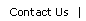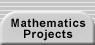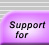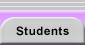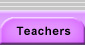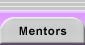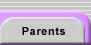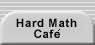# Logical Relationships Between Conditional Statements: The Converse, Inverse, and Contrapositive

Every Great American City Has At Least One College.
Worcester Has Ten. — Highway billboard in Worcester, MA

This billboard advertisement plays on the fact that people, in both daily life and within mathematics classes, tend to treat related, but logically distinct, conditional statements as equivalent. What is the advertisement trying to suggest? Is it supplying the necessary evidence?

A conditional statement is one that can be put in the form if A, then B where A is called the premise (or antecedent) and B is called the conclusion (or consequent). We can convert the above statement into this standard form: If an American city is great, then it has at least one college. The advertisers then share with us that Worcester has ten colleges, that is, that it satisfies the conclusion of the statement. They hope that we will then conclude that Worcester is great. Leaving aside the truth of that conclusion, it is not a logical deduction. Just because a premise implies a conclusion, that does not mean that the converse statement, if B, then A, must also be true. We do not need to accept the statement, "if an American city has at least one college, then it is great."

Why do we often fall into this trap (known as a converse error)? Because, everyday comments often carry an unstated second meaning. If we say "If it is raining, then I carry my umbrella," then people have some reason to assume the converse statement as well as the inverse (if not A, then not B), "if it is not raining, then I do not carry my umbrella." If these were not the case, you might just as well have said, "I carry my umbrella all of the time!" However, the truth of the inverse and converse of a statement are logically unrelated to the truth of the initial statement. Consider the true mathematical statement, "if a figure is a square, then it is a rectangle," which has false converse and inverse statements.

The Euler Diagram below represents the statement if A, then B. All of the points within the inner circle match the premise A. Because the circles are nested, those same points are within circle B (they possess the property associated with B). Notice that there are points in circle B that are not inside of circle A. Therefore, the converse statement, if B, then A, does not have to be true. If the converse were true, then circle B would need to be contained within A as well and the two circles would have to be identical. The same points that show that the converse might be false, also show that the inverse is suspect. There might be examples which do not have property A, but which do have property B so if not A, then not B is not dependable.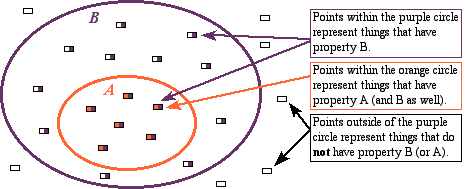The Euler Diagram for "if A, then B"The Euler Diagram for "if a figure is a triangle, then it is a polygon"

A third transformation of a conditional statement is the contrapositive, if not B, then not A. The contrapositive does have the same truth value as its source statement. The Euler diagram illustrates why the contrapositive is equivalent to the original statement. Because circle A is wholly within circle B, points outside of circle B (not B) must be outside of circle A (not A) as well. So not B implies not A. Note that the inverse and converse of the same statement are each other’s contrapositive and are, therefore, both true or both false conditional statements.

The equivalence of a statement and its contrapositive is at the heart of the method of proof by contradiction, which proves that the contrapositive of a conjecture is true and, therefore, that the original conjecture is true.

The first chapter of Harold Jacobs’s (1987) Geometry, 2nd Edition published by W. H. Freeman has a thorough discussion of these ideas with many good exercises.Translations of mathematical formulas for web display were created by tex4ht. © Copyright 2003 Education Development Center, Inc. (EDC)